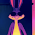## Friday, May 24, 2019

1.There was an explosion at the university of Lyon on January 17,2019 if it has anything to do with today’s explosion I’ve found they are 4 months and 7 days apart.

2."Explosion" = 444 S
"Hollywood" = 444 S <<<<<<<< Illuminati Card
"Kill Circle" = 444 S
"Agenda Title Codes" = 444 J
"Signal a Deception" = 444 J
"CIA Black Operation" = 444 J
"New World Order Government Agenda" = 444, 3333
"The Explosions on May Twenty Fourth" = 444 <<<<<<<<<<<<<<<<<<
"September Eleventh Two Thousand One Attack" = 444
"Do what thou Wilt Shall be the Whole of the Law" = 444

"Date Signal Digits" = 204 K
"Explosion in Lyon" = 204 K
"August Eleventh" = 204 K

"May Twenty Fourth" = 72
"August Eleventh" = 72

"City of Lyon France" = 191, 221 <<<< Previous News

"City of Lyon Explosions" = 103, 274
"August Eleventh Symbol" = 103, 274
"N.W.O. Police State Control" = 274
"Impending Tsunami Symbol" = 274
"Symbolic Nine One One" = 274
"Terror Attack Signal" = 274
"Coded Suicide Bomber" = 274

"Big Lebowski Code" = 666 S
"Victor Hugo Road" = 666 S
"Symbolic Code" = 666 J
"Prophecy" = 666 J

"Jeffery Lebowski Tribute" = 266
"August Eleventh Symbol" = 266

Write Out 266:

"Two Hundred Sixty Six" = 911 S
"Two Thousand Nineteen" = 911 S
"World Jewish Congress" = 911 S
"Symbol of Freemasonry" = 911 S
"Reverse Digits Symbol"" = 911 S
"Coded Protocol System" = 911 S
"The Big Lebowski is Here" = 911 S
"Donny Comes back to Life" = 911 S <<<<<<<<<<<<<<<<<<<<<<<<

^^^ Watch the First Few Minutes ^^^

"Big Lebowski Date Symbol" = 274 K
"August Eleventh Symbol " =274 K

Movie Released on March 6th:

"The Big Lebowski Movie Release Date" = 306 <<< March 6th?

"Donny's Return on Tisha Bav" = 274 K
"Comes back to Life Dates" = 274 K
"The Date Donny Returns" = 274 K
"August Eleventh Symbol" = 274 K

"Donny's Code" = 118 O <<<<